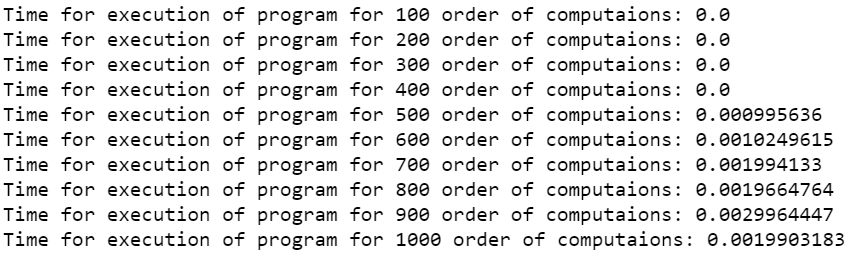Related Articles

# How to check the execution time of Python script ?

• Difficulty Level : Easy
• Last Updated : 16 Jul, 2021

In this article, we will discuss how to measure the running time of a Python Script.

There are many python modules like time, timeit, and datetime in Python which can store the time at which the particular section of the program is being executed. By manipulating or getting the difference between times of beginning and ending at which a particular section is being executed we can calculate the time it took to execute the section.

The following methods can be used to compute time difference:

• Time module in Python provides various time-related functions. This module comes under Python’s standard utility modules. time.time() method of Time module is used to get the time in seconds since epoch. The handling of leap seconds is platform-dependent.
• Python datetime library defines a function that can be primarily used to get the current time and date. now() function Return the current local date and time, which is defined under datetime module.
• timeit runs your snippet of code millions of times (default value is 1000000) so that you get the statistically most relevant measurement of code execution time.

Example 1: Computing the time using time module and time.time() function

## Python3

 `# program to compute the time``# of execution of any python code``import` `time` `# we initialize the variable start``# to store the starting time of``# execution of program``start ``=` `time.time()` `# we can take any program but for``# example we have taken the below``# program``a ``=` `0``for` `i ``in` `range``(``1000``):``    ``a ``+``=` `(i``*``*``100``)` `# now we have initialized the variable``# end to store the ending time after``# execution of program``end ``=` `time.time()` `# difference of start and end variables``# gives the time of execution of the``# program in between``print``(``"The time of execution of above program is :"``, end``-``start)`

Output:

`The time of execution of above program is : 0.001995563507080078`

We have computed the time of the above program which came out of the order 10^-3. We can check for the time by increasing the number of computations using the same algorithms.

Example 2: Checking times for execution of the program for different numbers of computations.

## Python3

 `# program to compute the time``# of execution of any python code``# for different number of computations``import` `time` `# we initialize a for loop and in each``# iterations store the time of start``# and end of the iterations``for` `j ``in` `range``(``100``, ``1100``, ``100``):``    ``start ``=` `time.time()` `    ``# program to iterate the range of``    ``# below loop increasing the value``    ``# in each iterations``    ``a ``=` `0``    ``for` `i ``in` `range``(j):``        ``a ``+``=` `(i``*``*``100``)` `    ``# the end variable to store the``    ``# ending time after execution of``    ``# program after each iterations``    ``end ``=` `time.time()` `    ``# difference of start and end variables``    ``# gives the time of execution of the program``    ``# in between in each iterations``    ``print``(``"Time for execution of program for {} order of computations: {}"``.``format``(``        ``j, ``round``(end``-``start, ``10``)))`

Output:We see a general trend in the increase in time of computation for an increase in the number of execution. However, it may not show any linear trend or fixed increments.

Example 3: Using datetime module in python and datetime.now function.

## Python3

 `# program to compute the time of``# execution of any python code``from` `datetime ``import` `datetime` `# we initialize the variable start to``# store the starting time of execution``# of program``start ``=` `datetime.now()` `# we can take any program but for``# example we have taken the below``# program``a ``=` `0``for` `i ``in` `range``(``1000``):``    ``a ``+``=` `(i``*``*``100``)` `# now we have initialized the variable``# end to store the ending time after``# execution of program``end ``=` `datetime.now()` `# difference of start and end variables``# gives the time of execution of the``# program in between``print``(``"The time of execution of above program is :"``,``      ``str``(end``-``start)[``5``:])`

Output:

`The time of execution of above program is : 00.001996`

Example 4: Using timeit

This would give us the execution time of any program. This module provides a simple way to find the execution time of small bits of Python code. It provides the timeit() method to do the same. The module function timeit.timeit(stmt, setup, timer, number) accepts four arguments:

• stmt which is the statement you want to measure; it defaults to ‘pass’.
• setup which is the code that you run before running the stmt; it defaults to ‘pass’. We generally use this to import the required modules for our code.
• timer which is a timeit.Timer object; it usually has a sensible default value so you don’t have to worry about it.
• number which is the number of executions you’d like to run the stmt.

## Python3

 `# program to compute the time of``# execution of any python code using timit` `# importing the required module``import` `timeit` `# code snippet to be executed only once``mysetup ``=` `"from math import sqrt"` `# code snippet whose execution time``# is to be measured``mycode ``=` `'''``def example():``    ``mylist = []``    ``for x in range(100):``        ``mylist.append(sqrt(x))``'''` `# timeit statement``print` `(``"The time of execution of above program is :"``,``       ``timeit.timeit(setup ``=` `mysetup,``                    ``stmt ``=` `mycode,``                    ``number ``=` `10000``))`

Output:

`The time of execution of above program is : 0.0023286999994525104`

Attention geek! Strengthen your foundations with the Python Programming Foundation Course and learn the basics.

To begin with, your interview preparations Enhance your Data Structures concepts with the Python DS Course. And to begin with your Machine Learning Journey, join the Machine Learning – Basic Level Course

My Personal Notes arrow_drop_up